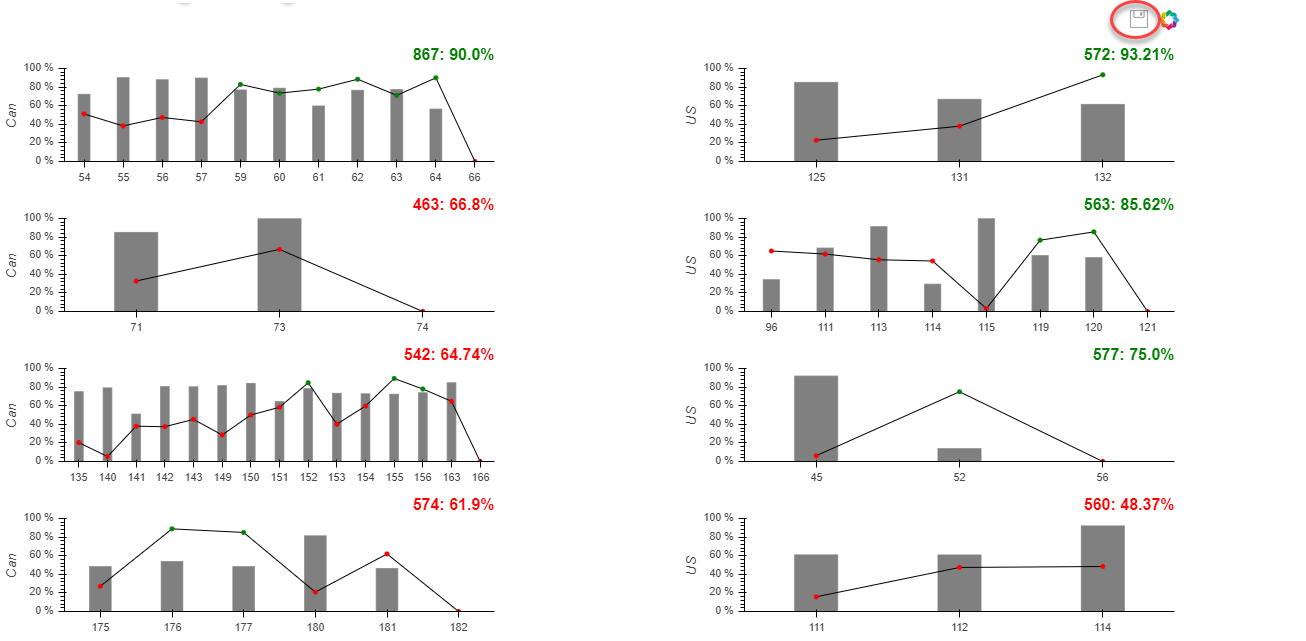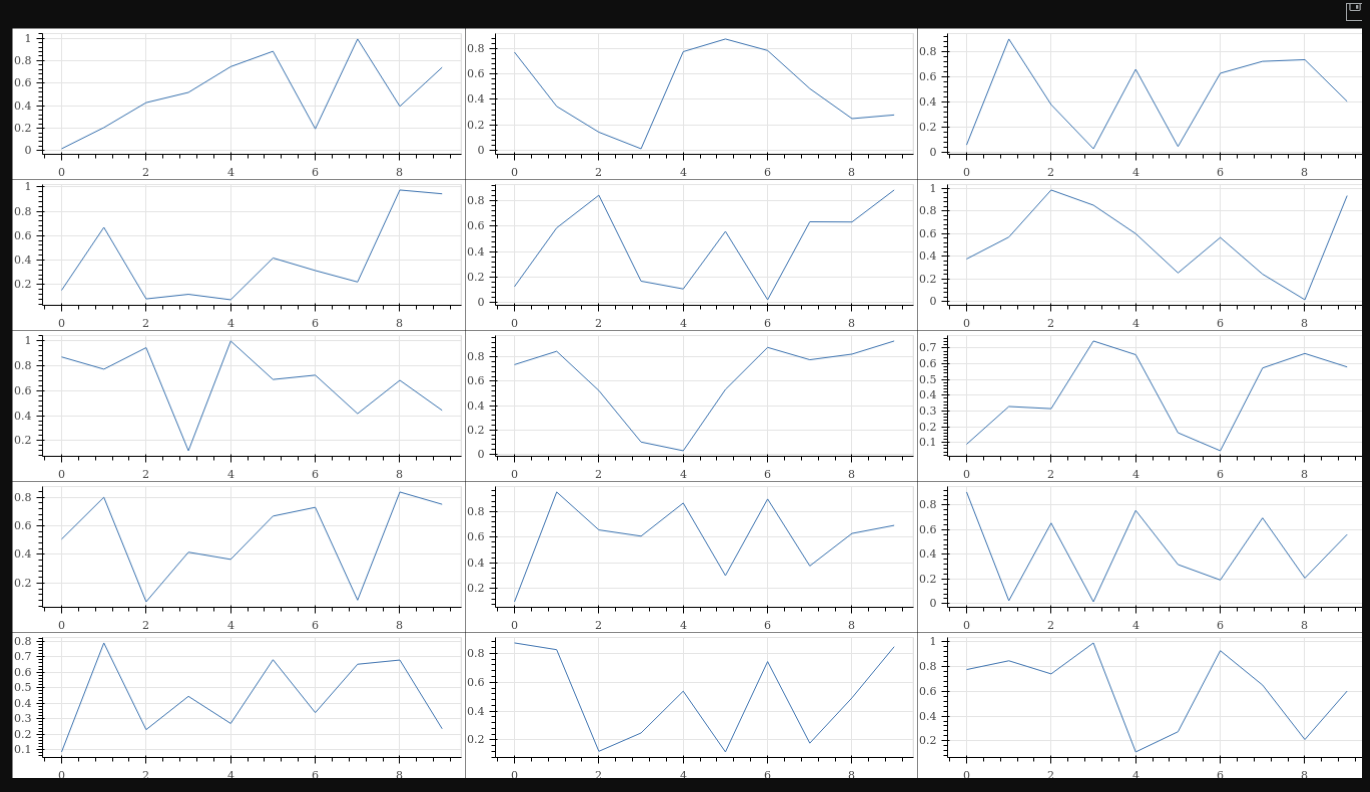# multiple plots share same "save"

Hi guys,

if I try to share “save” tool across multiple plots, how could I could do? thanksmy code like this:

TOOLS = [SaveTool(), HoverTool()]

rig_plot1 = figure(x_range = FactorRange(), \

plot_width = 500, \

plot_height = 150, \

tools = TOOLS)
rig_plot2 = figure(x_range = FactorRange(), \

plot_width = 500, \

plot_height = 150, \

tools = TOOLS)
rig_plot3 = figure(x_range = FactorRange(), \

plot_width = 500, \

plot_height = 150, \

tools = TOOLS)

``

It can not work as I expected. but, this solution can allow me to save plot one by one

Welcome any clue, thank.

BTW, every plot has their own specific datasource.

···

On Monday, February 11, 2019 at 11:00:20 AM UTC-7, peng wang wrote:

Hi guys,

if I try to share “save” tool across multiple plots, how could I could do? thanks

my code like this:

TOOLS = [SaveTool(), HoverTool()]

rig_plot1 = figure(x_range = FactorRange(), \

plot_width = 500, \

plot_height = 150, \

tools = TOOLS)
rig_plot2 = figure(x_range = FactorRange(), \

plot_width = 500, \

plot_height = 150, \

tools = TOOLS)
rig_plot3 = figure(x_range = FactorRange(), \

plot_width = 500, \

plot_height = 150, \

tools = TOOLS)

``

It can not work as I expected. but, this solution can allow me to save plot one by one

Welcome any clue, thank.

Hi,

This is not currently possible. There is an open feature request issue you can follow:

Thanks,

Bryan

···

On Feb 11, 2019, at 10:00, peng wang <[email protected]> wrote:

Hi guys,

if I try to share "save" tool across multiple plots, how could I could do? thanks

<2019-02-11_10-54-41.png>

my code like this:

TOOLS = [SaveTool(), HoverTool()]

rig_plot1 = figure(x_range = FactorRange(), \
plot_width = 500, \
plot_height = 150, \
tools = TOOLS)
rig_plot2 = figure(x_range = FactorRange(), \
plot_width = 500, \
plot_height = 150, \
tools = TOOLS)
rig_plot3 = figure(x_range = FactorRange(), \
plot_width = 500, \
plot_height = 150, \
tools = TOOLS)

It can not work as I expected. but, this solution can allow me to save plot one by one

Welcome any clue, thank.

--
You received this message because you are subscribed to the Google Groups "Bokeh Discussion - Public" group.
To unsubscribe from this group and stop receiving emails from it, send an email to [email protected].
To post to this group, send email to [email protected].
<2019-02-11_10-54-41.png>

good to know, If I try to print this plot, how could I do then? thanks

···

On Monday, February 11, 2019 at 11:05:13 AM UTC-7, Bryan Van de ven wrote:

Hi,

This is not currently possible. There is an open feature request issue you can follow:

``````    [https://github.com/bokeh/bokeh/issues/8531](https://github.com/bokeh/bokeh/issues/8531)
``````

Thanks,

Bryan

On Feb 11, 2019, at 10:00, peng wang [email protected] wrote:

Hi guys,

if I try to share “save” tool across multiple plots, how could I could do? thanks

<2019-02-11_10-54-41.png>

my code like this:

TOOLS = [SaveTool(), HoverTool()]

rig_plot1 = figure(x_range = FactorRange(), \

``````                      plot_width = 500, \
``````
``````                      plot_height = 150, \
``````
``````                      tools = TOOLS)
``````

rig_plot2 = figure(x_range = FactorRange(), \

``````                      plot_width = 500, \
``````
``````                      plot_height = 150, \
``````
``````                      tools = TOOLS)
``````

rig_plot3 = figure(x_range = FactorRange(), \

``````                      plot_width = 500, \
``````
``````                      plot_height = 150, \
``````
``````                      tools = TOOLS)
``````

It can not work as I expected. but, this solution can allow me to save plot one by one

Welcome any clue, thank.

You received this message because you are subscribed to the Google Groups “Bokeh Discussion - Public” group.

To unsubscribe from this group and stop receiving emails from it, send an email to [email protected].

To post to this group, send email to [email protected].

<2019-02-11_10-54-41.png>

Hi,

Bokeh's Python API function export_png can grab an entire document in one PNG, including grid plots. Otherwise I think you would have to look at coordinating Selenium and a headless browser to capture an image (which is all export_png does under the covers, anyway).

I can't think of any current way to be able to do this from within the browser, i.e. with a plot tool.

THanks,

Bryan

···

On Feb 11, 2019, at 10:15, peng wang <[email protected]> wrote:

good to know, If I try to print this plot, how could I do then? thanks

On Monday, February 11, 2019 at 11:05:13 AM UTC-7, Bryan Van de ven wrote:
Hi,

This is not currently possible. There is an open feature request issue you can follow:

Thanks,

Bryan

> On Feb 11, 2019, at 10:00, peng wang <[email protected]> wrote:
>
> Hi guys,
>
> if I try to share "save" tool across multiple plots, how could I could do? thanks
>
> <2019-02-11_10-54-41.png>
>
>
>
> my code like this:
>
>
> TOOLS = [SaveTool(), HoverTool()]
>
> rig_plot1 = figure(x_range = FactorRange(), \
> plot_width = 500, \
> plot_height = 150, \
> tools = TOOLS)
> rig_plot2 = figure(x_range = FactorRange(), \
> plot_width = 500, \
> plot_height = 150, \
> tools = TOOLS)
> rig_plot3 = figure(x_range = FactorRange(), \
> plot_width = 500, \
> plot_height = 150, \
> tools = TOOLS)
>
>
>
>
> It can not work as I expected. but, this solution can allow me to save plot one by one
>
>
>
> Welcome any clue, thank.
>
>
>
>
> --
> You received this message because you are subscribed to the Google Groups "Bokeh Discussion - Public" group.
> To unsubscribe from this group and stop receiving emails from it, send an email to [email protected].
> To post to this group, send email to [email protected].
> For more options, visit https://groups.google.com/a/continuum.io/d/optout.
> <2019-02-11_10-54-41.png>

--
You received this message because you are subscribed to the Google Groups "Bokeh Discussion - Public" group.
To unsubscribe from this group and stop receiving emails from it, send an email to [email protected].
To post to this group, send email to [email protected].
To view this discussion on the web visit https://groups.google.com/a/continuum.io/d/msgid/bokeh/e52621ee-8b55-4082-9d8f-d0a0d11fbbe3%40continuum.io.

The following code works just fine using the export_png() method:

import numpy as np
from bokeh.layouts import gridplot
from bokeh.plotting import figure, show
from bokeh.io import export_png

plots = [figure(plot_height = 200, tools = ‘save’) for i in range(15)]
[plot.line(np.arange(10), np.random.random(10)) for plot in plots]
all = gridplot(plots, merge_tools = True, ncols = 3, sizing_mode = ‘scale_width’, toolbar_options = dict(logo = None))
export_png(all, filename = “gridplot_new.png”)
show(all)

``

Bokeh info:

Python version : 3.5.1 (default, Feb 1 2019, 21:29:47)
IPython version : (not installed)
Bokeh version : 1.0.4
BokehJS static path : /usr/local/lib/python3.5/site-packages/bokeh/server/static
node.js version : v6.16.0
npm version : 3.10.10

``···

On Monday, February 11, 2019 at 8:25:34 PM UTC+1, Bryan Van de ven wrote:

Hi,

Bokeh’s Python API function export_png can grab an entire document in one PNG, including grid plots. Otherwise I think you would have to look at coordinating Selenium and a headless browser to capture an image (which is all export_png does under the covers, anyway).

I can’t think of any current way to be able to do this from within the browser, i.e. with a plot tool.

THanks,

Bryan

On Feb 11, 2019, at 10:15, peng wang [email protected] wrote:

good to know, If I try to print this plot, how could I do then? thanks

On Monday, February 11, 2019 at 11:05:13 AM UTC-7, Bryan Van de ven wrote:

Hi,

This is not currently possible. There is an open feature request issue you can follow:

``````    [https://github.com/bokeh/bokeh/issues/8531](https://github.com/bokeh/bokeh/issues/8531)
``````

Thanks,

Bryan

On Feb 11, 2019, at 10:00, peng wang [email protected] wrote:

Hi guys,

if I try to share “save” tool across multiple plots, how could I could do? thanks

<2019-02-11_10-54-41.png>

my code like this:

TOOLS = [SaveTool(), HoverTool()]

rig_plot1 = figure(x_range = FactorRange(),
plot_width = 500,
plot_height = 150,
tools = TOOLS)
rig_plot2 = figure(x_range = FactorRange(),
plot_width = 500,
plot_height = 150,
tools = TOOLS)
rig_plot3 = figure(x_range = FactorRange(),
plot_width = 500,
plot_height = 150,
tools = TOOLS)

It can not work as I expected. but, this solution can allow me to save plot one by one

Welcome any clue, thank.

You received this message because you are subscribed to the Google Groups “Bokeh Discussion - Public” group.
To unsubscribe from this group and stop receiving emails from it, send an email to [email protected].
To post to this group, send email to [email protected].
<2019-02-11_10-54-41.png>

You received this message because you are subscribed to the Google Groups “Bokeh Discussion - Public” group.

To unsubscribe from this group and stop receiving emails from it, send an email to [email protected].

To post to this group, send email to [email protected].

To view this discussion on the web visit https://groups.google.com/a/continuum.io/d/msgid/bokeh/e52621ee-8b55-4082-9d8f-d0a0d11fbbe3%40continuum.io.

You may also need:

# selenium 3.141.0

``

···

On Tuesday, February 12, 2019 at 2:23:10 PM UTC+1, tony halik wrote:

The following code works just fine using the export_png() method:

import numpy as np
from bokeh.layouts import gridplot
from bokeh.plotting import figure, show
from bokeh.io import export_png

plots = [figure(plot_height = 200, tools = ‘save’) for i in range(15)]
[plot.line(np.arange(10), np.random.random(10)) for plot in plots]
all = gridplot(plots, merge_tools = True, ncols = 3, sizing_mode = ‘scale_width’, toolbar_options = dict(logo = None))
export_png(all, filename = “gridplot_new.png”)
show(all)

``

Bokeh info:

Python version : 3.5.1 (default, Feb 1 2019, 21:29:47)
IPython version : (not installed)
Bokeh version : 1.0.4
BokehJS static path : /usr/local/lib/python3.5/site-packages/bokeh/server/static
node.js version : v6.16.0
npm version : 3.10.10

``

On Monday, February 11, 2019 at 8:25:34 PM UTC+1, Bryan Van de ven wrote:

Hi,

Bokeh’s Python API function export_png can grab an entire document in one PNG, including grid plots. Otherwise I think you would have to look at coordinating Selenium and a headless browser to capture an image (which is all export_png does under the covers, anyway).

I can’t think of any current way to be able to do this from within the browser, i.e. with a plot tool.

THanks,

Bryan

On Feb 11, 2019, at 10:15, peng wang [email protected] wrote:

good to know, If I try to print this plot, how could I do then? thanks

On Monday, February 11, 2019 at 11:05:13 AM UTC-7, Bryan Van de ven wrote:

Hi,

This is not currently possible. There is an open feature request issue you can follow:

``````    [https://github.com/bokeh/bokeh/issues/8531](https://github.com/bokeh/bokeh/issues/8531)
``````

Thanks,

Bryan

On Feb 11, 2019, at 10:00, peng wang [email protected] wrote:

Hi guys,

if I try to share “save” tool across multiple plots, how could I could do? thanks

<2019-02-11_10-54-41.png>

my code like this:

TOOLS = [SaveTool(), HoverTool()]

rig_plot1 = figure(x_range = FactorRange(),
plot_width = 500,
plot_height = 150,
tools = TOOLS)
rig_plot2 = figure(x_range = FactorRange(),
plot_width = 500,
plot_height = 150,
tools = TOOLS)
rig_plot3 = figure(x_range = FactorRange(),
plot_width = 500,
plot_height = 150,
tools = TOOLS)

It can not work as I expected. but, this solution can allow me to save plot one by one

Welcome any clue, thank.

You received this message because you are subscribed to the Google Groups “Bokeh Discussion - Public” group.
To unsubscribe from this group and stop receiving emails from it, send an email to [email protected].
To post to this group, send email to [email protected].